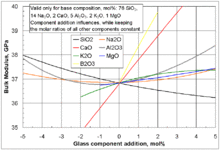# Bulk modulus

Bulk modulusIllustration of uniform compression

The bulk modulus (K) of a substance measures the substance's resistance to uniform compression. It is defined as the pressure increase needed to decrease the volume by a factor of 1/e. Its base unit is the pascal.

## Definition

The bulk modulus K>0 can be formally defined by the equation:$K=-V\frac{\partial P}{\partial V}$

where P is pressure, V is volume, and ∂P/∂V denotes the partial derivative of pressure with respect to volume. The inverse of the bulk modulus gives a substance's compressibility.

Other moduli describe the material's response (strain) to other kinds of stress: the shear modulus describes the response to shear, and Young's modulus describes the response to linear stress. For a fluid, only the bulk modulus is meaningful. For an anisotropic solid such as wood or paper, these three moduli do not contain enough information to describe its behaviour, and one must use the full generalized Hooke's law.

## Thermodynamic relation

Strictly speaking, the bulk modulus is a thermodynamic quantity, and it is necessary to specify how the temperature varies in order to specify a bulk modulus: constant-temperature (isothermal KT), constant-entropy (adiabatic KS), and other variations are possible. In practice, such distinctions are usually only relevant for gases.

For a gas, the adiabatic bulk modulus KS is approximately given by$K_S=\gamma\, P$

and the isothermal bulk modulus KT is approximately given by$K_T=P\,$

where

γ is the adiabatic index, sometimes called κ.
P is the pressure.

In a fluid, the bulk modulus K and the density ρ determine the speed of sound c (pressure waves), according to the Newton-Laplace formula$c=\sqrt{\frac{K}{\rho}}.$

Solids can also sustain transverse waves: for these materials one additional elastic modulus, for example the shear modulus, is needed to determine wave speeds.

## Measurement

It is possible to measure the bulk modulus using powder diffraction under applied pressure.

## Selected values

Approximate bulk modulus (K) for common materials
Material Bulk modulus in GPa Bulk modulus in ksi
Steel 160 23×103
Diamond 442 64×103Influences of selected glass component additions on the bulk modulus of a specific base glass.

Material with bulk modulus value of 35GPa needs external pressure of 0.35 GPa (~3500Bar) to reduce the volume by one percent.

 Water 2.2×109 Pa (value increases at higher pressures) Air 1.42×105 Pa (adiabatic bulk modulus) Air 1.01×105 Pa (constant temperature bulk modulus) Solid helium 5×107 Pa (approximate)

Wikimedia Foundation. 2010.

### Look at other dictionaries:

• bulk modulus — Physics. a coefficient of elasticity of a substance, expressing the ratio between a pressure that acts to change the volume of the substance and the fractional change in volume produced. [1905 10] * * * Numerical constant that describes the… …   Universalium

• bulk modulus — spūdos modulis statusas T sritis Standartizacija ir metrologija apibrėžtis Dydis, išreiškiamas kūną veikiančio slėgio ir santykinės jo tūrio deformacijos dalmeniu visapusio suslėgimo ar tempimo atveju, t. y. K = p/ϑ; čia p – slėgis, ϑ – santykinė …   Penkiakalbis aiškinamasis metrologijos terminų žodynas

• bulk modulus — spūdos modulis statusas T sritis fizika atitikmenys: angl. bulk modulus; compressibility modulus vok. Kompressionsmodul, m; Volumenelastizitätsmodul, m rus. модуль объёмного сжатия, m; модуль объёмной упругости, m pranc. module de compressibilité …   Fizikos terminų žodynas

• bulk modulus — noun the ratio of the change in pressure acting on a volume to the fractional change in volume • Hypernyms: ↑coefficient of elasticity, ↑modulus of elasticity, ↑elastic modulus * * * noun : the ratio of the intensity of stress to the volume… …   Useful english dictionary

• bulk modulus — /bʌlk ˈmɒdʒələs/ (say bulk mojuhluhs) noun Physics the ratio of the volume of a body to the pressure distributed uniformly over the whole of its surface …   Australian-English dictionary

• bulk modulus — noun Physics the relative change in the volume of a body produced by a unit compressive or tensile stress acting uniformly over its surface …   English new terms dictionary

• Bulk modulus of elasticity (К) — Bulk modulus of elasticity (К). См. Объемный модуль упругости (К). (Источник: «Металлы и сплавы. Справочник.» Под редакцией Ю.П. Солнцева; НПО Профессионал , НПО Мир и семья ; Санкт Петербург, 2003 г.) …   Словарь металлургических терминов

• Bulk — can refer to:*Bulk mail *Bulk purchasing *Bulk liquids *Bulk modulus *Bulk material handling *Bulk British professional wrestler * Bulk and Skull, a pair of characters in the Power Rangers universe. * The bulk in brane cosmology is a hypothetical …   Wikipedia

• modulus of elasticity — noun (physics) the ratio of the applied stress to the change in shape of an elastic body • Syn: ↑coefficient of elasticity, ↑elastic modulus • Topics: ↑physics, ↑natural philosophy • Hypernyms: ↑ …   Useful english dictionary

• modulus of compression — spūdos modulis statusas T sritis Standartizacija ir metrologija apibrėžtis Dydis, išreiškiamas kūną veikiančio slėgio ir santykinės jo tūrio deformacijos dalmeniu visapusio suslėgimo ar tempimo atveju, t. y. K = p/ϑ; čia p – slėgis, ϑ – santykinė …   Penkiakalbis aiškinamasis metrologijos terminų žodynas